来聊聊 SwiftUI 中的 Text 插值和本地化 (下)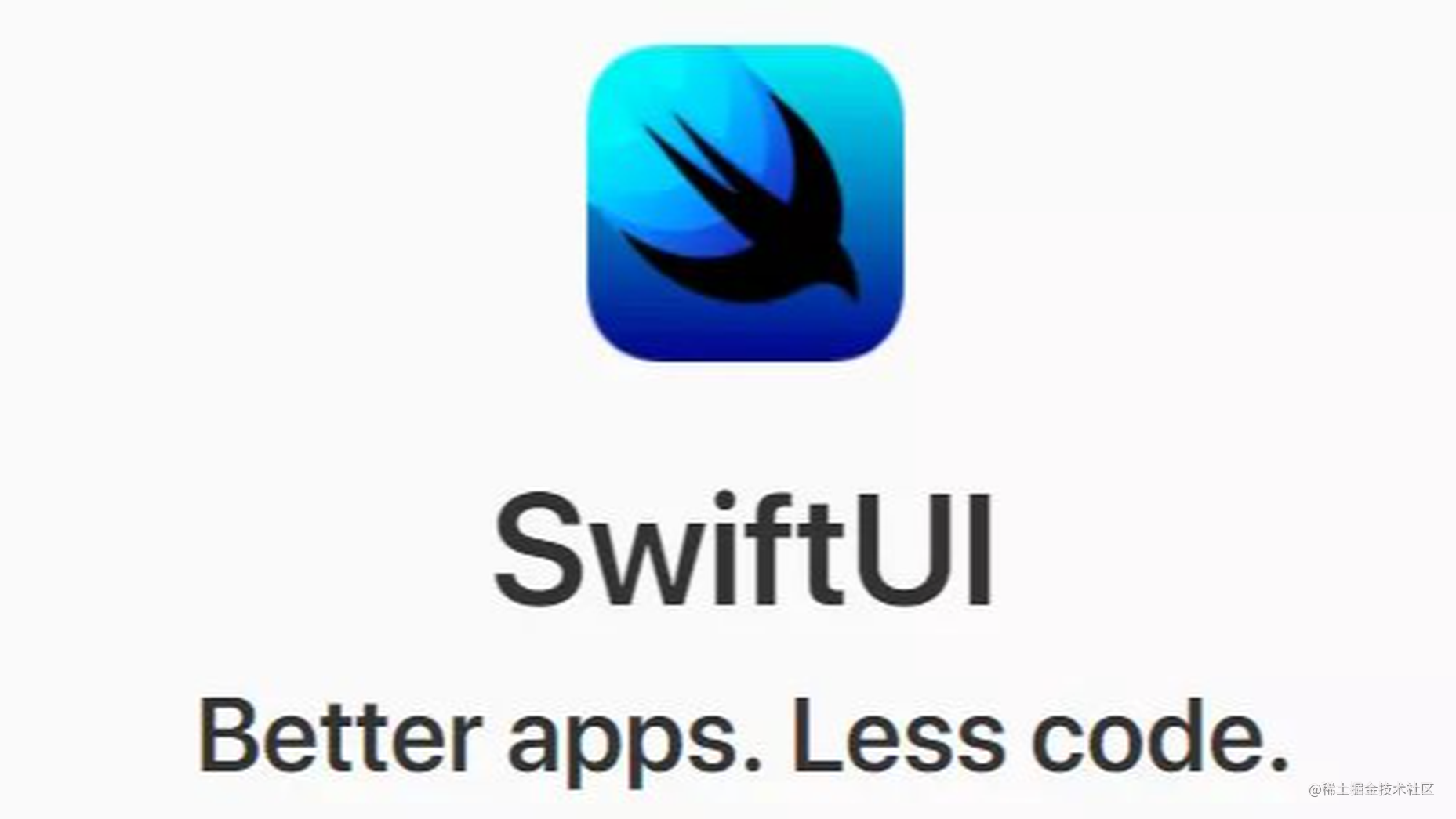为 LocalizedStringKey 自定义插值

extension LocalizedStringKey.StringInterpolation {
mutating func appendInterpolation(_ value: Bool) {
appendLiteral(value.description)
}
}

Text("3 == 3 is (true)")

extension LocalizedStringKey.StringInterpolation {
mutating func appendInterpolation(_ person: Person, isFriend: Bool) {
appendLiteral(person.title(isFriend: isFriend))
}
}

LocalizedStringKey 的真面目

通过 key 查找本地化值

let key1: LocalizedStringKey = "Hello World"
print(key1)
// LocalizedStringKey(
//     key: "Hello World",
//     hasFormatting: false,
//     arguments: []
// )

// Localization.strings
"Hello World"="你好，世界";

Text("Hello World")
Text("Hello World")
.environment(.locale, Locale(identifier: "zh-Hans"))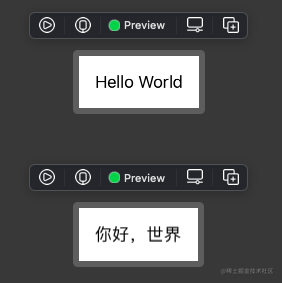插值 LocalizedStringKey 的 key

let name = "onevcat"
let key2: LocalizedStringKey = "I am (name)"

print(key2)

// LocalizedStringKey(
//     key: "I am %@",
//     hasFormatting: true,
//     arguments: [
//         SwiftUI.LocalizedStringKey.FormatArgument(
//             ...storage: Storage.value("onevcat", nil)
//         )
//     ]
// )

key 并不是固定的 “I am onevcat”，而是一个 String formatter：”I am %@”。熟悉 String format 的读者肯定对此不会陌生：name 被作为变量，会被传递到 String format 中，并替换掉 %@ 这个表示对象的占位符。所以，在本地化这个字符串的时候，我们需要指定的 key 是 “I am %@”。当然，这个 LocalizedStringKey 也可以对应其他任意的输入：

// Localization.strings
"I am %@"="我是%@";

// ContentView.swift
Text("I am ("onevcat")")
// 我是onevcat

Text("I am ("张三")")
// 我是张三

let key3: LocalizedStringKey = "Hello (Image(systemName: "globe"))"

print(key3)
// LocalizedStringKey(
//     key: "Hello %@",
//     ...
// )

// Localization.strings
// "Hello %@"="你好，%@";

Text("Hello (Image(systemName: "globe"))")
Text("Hello (Image(systemName: "globe"))")
.environment(.locale, Locale(identifier: "zh-Hans"))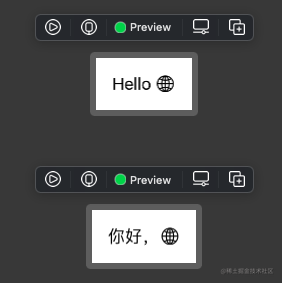Text("Hello ("onevcat")")
.environment(.locale, Locale(identifier: "zh-Hans"))
Text("Hello (Image(systemName: "globe"))")
.environment(.locale, Locale(identifier: "zh-Hans"))

其他类型的插值格式化

let key4: LocalizedStringKey = "Hello (1))"
// LocalizedStringKey(key: "Hello %lld)

let key5: LocalizedStringKey = "Hello (1.0))"
// LocalizedStringKey(key: "Hello %lf)

更合理的 appendInterpolation 实现

避免 appendLiteral

mutating func appendInterpolation(_ value: Bool) {
appendLiteral(value.description)
}

mutating func appendInterpolation(_ person: Person, isFriend: Bool) {
appendLiteral(person.title(isFriend: isFriend))
}

let key6: LocalizedStringKey = "3 == 3 is (true)"
// LocalizedStringKey(key: "3 == 3 is true", ...)

let person = Person(name: "Geralt", place: "Rivia", nickName: "White Wolf")
let key7: LocalizedStringKey = "Hi, (person, isFriend: false)"
// LocalizedStringKey(key: "Hi, Geralt of Rivia", ...)

mutating func appendInterpolation(_ value: Bool) {
appendInterpolation(value.description)
}

mutating func appendInterpolation(_ person: Person, isFriend: Bool) {
appendInterpolation(person.title(isFriend: isFriend))
}

let key6: LocalizedStringKey = "3 == 3 is (true)"
// LocalizedStringKey(key: "3 == 3 is %@", ...)

let key7: LocalizedStringKey = "Hi, (person, isFriend: false)"
// LocalizedStringKey(key: "Hi, %@", ...)

为 Text 添加样式

extension LocalizedStringKey.StringInterpolation {
mutating func appendInterpolation(bold value: LocalizedStringKey){
appendInterpolation(Text(value).bold())
}

mutating func appendInterpolation(underline value: LocalizedStringKey){
appendInterpolation(Text(value).underline())
}

mutating func appendInterpolation(italic value: LocalizedStringKey) {
appendInterpolation(Text(value).italic())
}

mutating func appendInterpolation(_ value: LocalizedStringKey, color: Color?) {
appendInterpolation(Text(value).foregroundColor(color))
}
}

Text("A \(bold: "wonderful") serenity \(italic: "has taken") \("possession", color: .red) of my \(underline: "entire soul").")关于 _FormatSpecifiable

mutating func appendInterpolation<T>(_ value: T) where T : _FormatSpecifiable
mutating func appendInterpolation<T>(_ value: T, specifier: String) where T : _FormatSpecifiable

指定占位格式

Swift 中的部分基本类型，是满足 _FormatSpecifiable 这个私有协议的。该协议帮助 LocalizedStringKey 在拼接 key 时选取合适的占位符表示，比如对 Int 选取 %lld，对 Double 选取 %lf 等。当我们使用 Int 或 Double 做插值时，上面的重载方法将被使用：

Text("1.5 + 1.5 = (1.5 + 1.5)")

// let key: LocalizedStringKey = "1.5 + 1.5 = (1.5 + 1.5)"
// print(key)
// 1.5 + 1.5 = %lf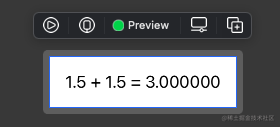Text("1.5 + 1.5 = (1.5 + 1.5, specifier: "%.1lf")")

// key: 1.5 + 1.5 = %.1lf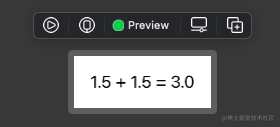为自定义类型实现 _FormatSpecifiable

protocol _FormatSpecifiable: Equatable {
associatedtype _Arg
var _arg: _Arg { get }
var _specifier: String { get }
}

extension Int: _FormatSpecifiable {
var _arg: Int { self }
var _specifier: String { "%lld" }
}

extension Person: _FormatSpecifiable {
var _arg: String { "(name) of (place)" }
var _specifier: String { "%@" }
}

总结

• 我们尝试扩展了 LocalizedStringKey 插值的方法，让它支持了 Bool 和 Person
• LocalizedStringKey 插值的主要任务是自动生成合适的，带有参数的本地化 key。
• 在扩展 LocalizedStringKey 插值时，应该是尽可能使用 appendInterpolation，避免参数“被吞”。
• 插值的格式是由 _FormatSpecifiable 确定的。我们也可以通过让自定义类型实现这个协议的方式，来进行插值。
• iOS各类技术资料下载

iOS